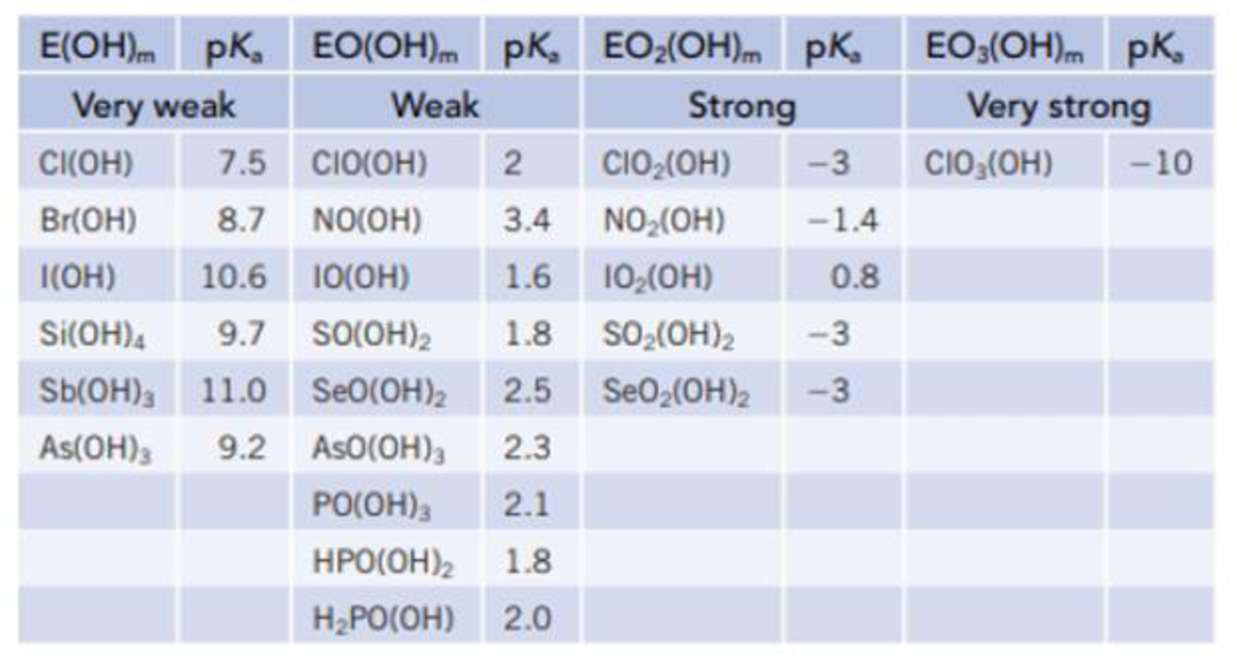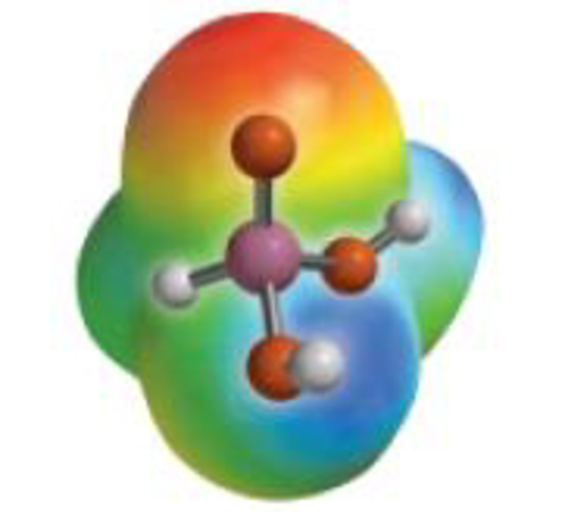Chapter 16, Problem 122SCQ

Chapter
Section
Textbook Problem

The acidity of the oxoacids was described in Section 16.9, and a larger number of acids are listed in the table below. (a) What general trends do you see in these data? (b) What has a greater effect on acidity, the number of O atoms bonded directly to the central atom E or the number of OH groups? (c) Look at the acids based on Cl, N, and S. Is there a correlation of acidity with the formal charge on the central atom, E?(d) The acid H3PO3 has a pKa of 1.8, and this led to some insight into its structure. If the structure of the acid were P(OH)3, what would be its predicted pKa value? Given that this is a diprotic acid, which H atoms are lost as H+ ions?The odd H3PO3

(a)

Interpretation Introduction

Interpretation:

General trend in given data has to be explained.

Concept Introduction:

From the data, the acidity increases with increase in electronegativity. Acidity increases down a group with an increase in atom size.

If pKa of an acid is more, its Ka will be less and it will be less acidic in nature.

If pKa of an acid is less, its Ka will be more and it will be more acidic.

For the same atom, if the formal charge on the central increases then the acidity increases.

Explanation

For very weak acids in column first, the value of pKa is highest among the given acids.

As the strength of acid changes from very weak acid to weak acid and followed by strong acid and very strong, the <

(b)

Interpretation Introduction

Interpretation:

The greater effect on acidity has to be explained when the oxygen atom is directly attached to central atom E or OH group.

Concept Introduction:

Acidity increases with increase in electronegativity. Acidity increases down a group with an increase in atom size.

If pKa of an acid is more, its Ka will be less and it will be less acidic in nature.

If pKa of an acid is less, its Ka will be more and it will be more acidic.

For the same atom, if the formal charge on the central increases then the acidity increases.

(c)

Interpretation Introduction

Interpretation:

It is stated whether the formal charge on the atoms Cl , S and N has an effect on the acidity of acids having these atoms as the central atom.

Concept Introduction:

Acidity increases with increase in electronegativity. Acidity increases down a group with an increase in atom size.

If pKa of an acid is more, its Ka will be less and it will be less acidic in nature.

If pKa of an acid is less, its Ka will be more and it will be more acidic.

For the same atom, if the formal charge on the central increases then the acidity increases.

(d)

Interpretation Introduction

Interpretation:

The value of pKa for H3PO3 has to be predicted when the structure is P(OH)3. The hydrogen has to be identified when losing as (H+) from diprotic acid.

Concept Introduction:

Acidity increases with increase in electronegativity. Acidity increases down a group with an increase in atom size.

If pKa of an acid is more, its Ka will be less and it will be less acidic in nature.

If pKa of an acid is less, its Ka will be more and it will be more acidic.

For the same atom, if the formal charge on the central increases then the acidity increases.

Still sussing out bartleby?

Check out a sample textbook solution.

See a sample solution

The Solution to Your Study Problems

Bartleby provides explanations to thousands of textbook problems written by our experts, many with advanced degrees!

Get Started